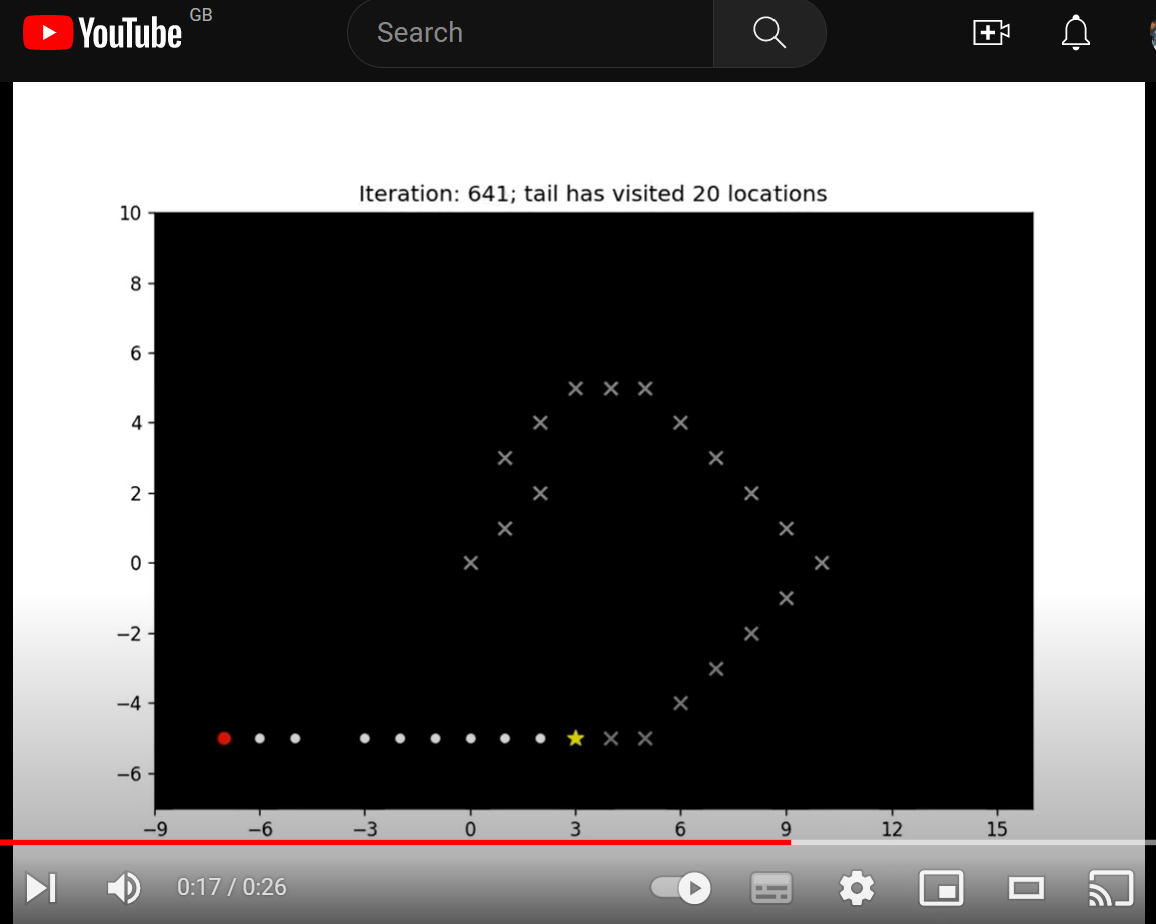# Learning Python with Advent of Code Walkthroughs

Dazbo's Advent of Code solutions, written in Python

# The Python Journey - Working with Images and Animations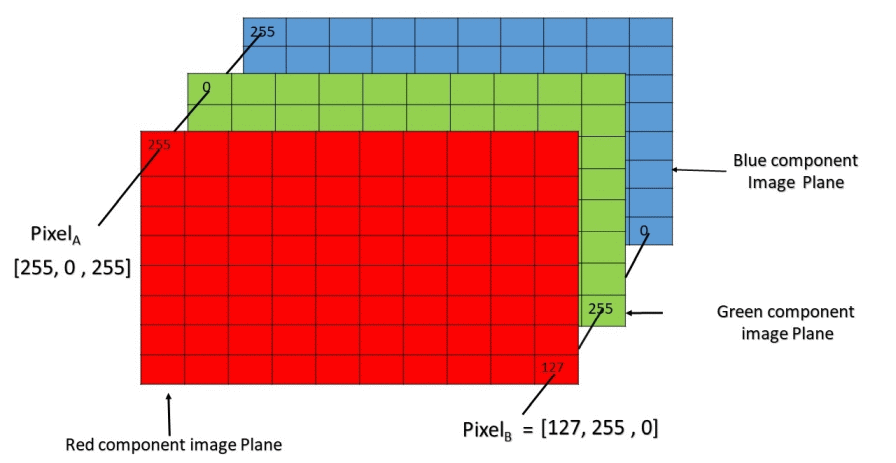## Overview

Images can be described as a two dimensional grid of pixels, where each pixel has a particular colour. For grayscale images, the pixels simply require intensity. Consequently, a grayscale image can be represented as a two dimensional ndarray, with shape (x, y). Whereas a colour image is typically represented as a two dimensional grid of pixels, plus three channels for each of R, G, B. Consequently, an RGB image can be represented as an ndarray with shape (x, y, 3).

There are a few ways to work with images in Python. These include:

• NumPy / Matplotlib
• Images are represented as ndarray objects: [x-size, y-size, colour channels]
• When Matplotlib is working with images, the underlying format is the NumPy ndarray.
• Pillow
• Extensive image format support and general image processing.
• Capabilities include image processing, thumbnail creation, conversion between formats, point operations, animations, and image transformation.
• ImageIO
• Useful package for reading and writing images.
• Particularly useful for animations, and for capturing images from various sources and streams.

## Working with Different Imaging Libraries

The code below demonstrates how to read and write images with a few Python libraries, and how to convert between them.

First, some basic imports and prep…

``````from os import path, mkdir
from io import BytesIO
import imageio as iio
from PIL import Image
from matplotlib import pyplot as plt, image as plt_img
import numpy as np

SCRIPT_DIR = path.abspath(path.curdir)
print(SCRIPT_DIR)
OUTPUT_DIR = path.join(SCRIPT_DIR, "output")
IMG_FILE = path.join(SCRIPT_DIR, "my_pic.jpg")

if not path.exists(OUTPUT_DIR):
mkdir(OUTPUT_DIR)
``````

``````print("Loading image with PIL...")
pil_image = Image.open(IMG_FILE)
print(f"Type: {type(pil_image)}")
print(f"Size: {pil_image.size}")
print(f"Format: {pil_image.format}")
print(f"Mode: {pil_image.mode}")
pil_image.show("Pillow Image")  # show the image
``````
``````Loading image with PIL...
Type: <class 'PIL.JpegImagePlugin.JpegImageFile'>
Size: (400, 225)
Format: JPEG
Mode: RGB
``````

``````print("\nLoading image with matplotlib...")
print(f"Type: {type(py_img)}")
print(f"Dtype: {py_img.dtype}")
print(f"Shape: {py_img.shape}")
plt.axis("off")
plt.imshow(py_img)  # attach the image to the plot
plt.show()  # show the image
``````
``````Loading image with matplotlib...
Type: <class 'numpy.ndarray'>
Dtype: uint8
Shape: (225, 400, 3)
``````

### Converting from Pillow to NumPy

``````print("\nConverting from Pillow to NumPy ndarray...")
from_pillow_to_numpy = np.array(pil_image)
print(f"Type: {type(from_pillow_to_numpy)}")
print(f"Dtype: {from_pillow_to_numpy.dtype}")
print(f"Shape: {from_pillow_to_numpy.shape}")
plt.axis("off")
plt.imshow(from_pillow_to_numpy)
plt.show()
``````
``````Converting from Pillow to NumPy ndarray...
Type: <class 'numpy.ndarray'>
Dtype: uint8
Shape: (225, 400, 3)
``````

### Converting from NumPy to Pillow

``````print("\nConverting from NumPy ndarray to Pillow...")
from_numpy_to_pillow = Image.fromarray(py_img)
print(f"Type: {type(from_numpy_to_pillow)}")
print(f"Size: {from_numpy_to_pillow.size}")
print(f"Format: {from_numpy_to_pillow.format}")
print(f"Mode: {from_numpy_to_pillow.mode}")
from_numpy_to_pillow.show("From NumPy to Pillow")
``````
``````Converting from NumPy ndarray to Pillow...
Type: <class 'PIL.Image.Image'>
Size: (400, 225)
Format: None
Mode: RGB
``````

### Converting from Matplotlib to BytesIO

``````print(f"Source type: {type(py_img)}")
frame = BytesIO()
plt.imshow(py_img)  # load the image into Plt
plt.savefig(frame, format='png')  # save the image to (BytesIO) memory
plt.savefig(Path(OUTPUT_DIR, "pyplot_img_file.png"))  # save to disk
``````

### Converting from BytesIO to Pillow

``````print("\nReading BytesIO in Pillow...")
pil_img = Image.open(frame) # Pillow open seeks for us
pil_img.show()
``````

### From Pillow to Various Formats, Including BytesIO

``````print("\nOpening image in Pillow and then saving in various formats to")
print(path.abspath(OUTPUT_DIR))
pil_img = Image.open(IMG_FILE)
pil_img.save(Path(OUTPUT_DIR, "pil_img_file.jpg"))
pil_img.save(Path(OUTPUT_DIR, "pil_img_file.png"))
print("And saving directly to BytesIO...")
frame = BytesIO()
pil_img.save(frame, format="PNG") # We can save to a file, or to a file-like object, like BytesIO
print("Success.")
``````

### From BytesIO to Pillow

``````print("\nOpening newly saved BytesIO in Pillow...")
pil_img = Image.open(frame) # Pillow open seeks for us
pil_img.show()
``````

### From BytesIO to Matplotlib

``````print("And reading the BytesIO back in using Pillow, and convert to Matplotlib...")
pil_img = Image.open(frame) # Pillow open seeks for us
plt_img = np.asarray(pil_image)
plt.imshow(plt_img)
plt.show()
``````

### From BytesIO to ImageIO

``````# From BytesIO into ImageIO
print("From BytesIO back into ImageIO...")
frame.seek(0)   # We need to seek back to 0
``````

### From ImageIO to File, and File to Pillow

``````# And saving ImageIO to file
print("From ImageIO to file...")
iio.imsave(Path(OUTPUT_DIR, "iio_file.png"), iio_img)

# Let's read it back, to prove...
print("And from file to Pillow to show...")
pil_img = Image.open(Path(OUTPUT_DIR, "iio_file.png")) # Pillow open seeks for us
pil_img.show()
``````

## Examples

### Heatmap

``````    def render_image(self, target_width:int=600) -> Image.Image:
""" Render grid as a heatmap image

Args:
width (int, optional): Target width, in pxiels. Defaults to 600.
"""
scale = target_width // self._width  # our original image is only a few pixels across. We need to scale up.

# Flatten our x,y array into a single list of height values
height_values = [self.height_at_point(Point(x,y)) for y in range(self._height)
for x in range(self._width)]
max_height = max(height_values)

# create a new list of RGB values, where each is given by an (R,G,B) tuple.
# To achieve a yellow->amber->red effect, we want R to always be 255, B to always be 0, and G to vary based on height
pixel_colour_map = list(map(lambda x: (255, int(255*((max_height-x)/max_height)), 0), height_values))

image = Image.new(mode='RGB', size=(self._width, self._height))
image.putdata(pixel_colour_map)  # load our colour map into the image

# scale the image and return it
return image.resize((self._width*scale, self._height*scale), Image.NEAREST)
``````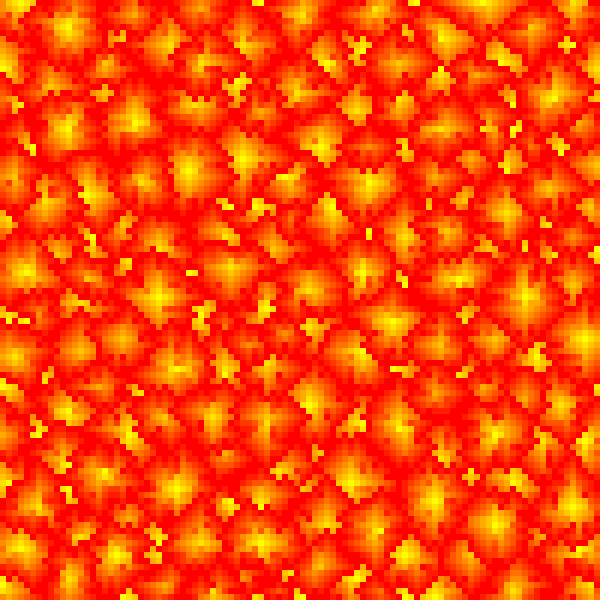### Flashing Grid

Taken from 2021 Day 11 - Flashing Octopi:

``````    def generate_frame(self):
""" Render an image frame showing the current cycle state.
Saves the frame to the self._frames list.
Superimposes the cycle number text to the frame. """
scale = 50
all_values = [self.value_at_point(point) for point in self.all_points()] # flattened values
max_energy = Grid.FLASH_THRESHOLD

# create a new list of pixels, where each is given by an (R,G,B) tuple.
pixel_colour_map = list(map(lambda x: (x*255//max_energy, 0, 0), all_values))

# Create an image from the flattened list of pixels, and scale up the size
small_image = Image.new(mode='RGB', size=(self.x_size, self.y_size))
small_image.putdata(pixel_colour_map)  # load our original data into the image
scaled_image = small_image.resize((self.x_size * scale, self.y_size * scale))

# Add our cycle count text to the bottom right of the image
image_draw = ImageDraw.Draw(scaled_image)
font = ImageFont.truetype('arial.ttf', 24)
text = str(self._generation)
rgba = (140, 200, 250, 255) # light blue
textwidth, textheight = image_draw.textsize(text, font)
im_width, im_height = scaled_image.size
margin = 10     # margin we want round the text to the edge
x_locn = im_width - textwidth - margin
y_locn = im_height - textheight - margin
image_draw.text((x_locn, y_locn), text, font=font, fill=rgba)

if (0, 0, 0) in pixel_colour_map:  # if 0 in colour_map, we need to flash
flash_image = small_image.copy()
new_image_data = []
for pixel in pixel_colour_map:
if pixel == (0, 0, 0):  # replace black with white
new_image_data.append((255, 255, 255))
else:
new_image_data.append(pixel)

# Add the new image data, and then resize as before
flash_image.putdata(new_image_data)
flash_image = flash_image.resize((self.x_size * scale, self.y_size * scale))

flash_image_draw = ImageDraw.Draw(flash_image)
flash_image_draw.text((x_locn, y_locn), text, font=font, fill=rgba)

# Flash frame needs to get appended before the 'black' frame
self._frames.append(flash_image)

self._frames.append(scaled_image)
``````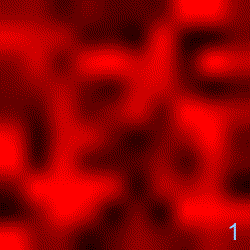### Trench Map

Taken from 2021 Day 20 - Trench Map Enhancement:

``````    def _render_image(self) -> Image.Image:
""" Render as an image """
width = (self._max_x+1) - self._min_x
height = (self._max_y+1) - self._min_y

image = Image.new(mode='RGB', size=(width, height))
image_data = []

for y in range(width):
for x in range(height):
x_val = x + self._min_x
y_val = y + self._min_y
point = Point(x_val, y_val)

if point in self._pixels:
image_data.append((255, 255, 255)) # lit pixels
else:
image_data.append((128, 0, 0)) # dark pixels

image.putdata(image_data)
return image
``````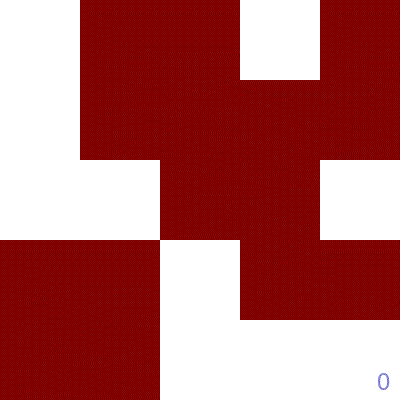### Height Map

Taken from 2022 Day 8 - Tree House Grid:

``````    def render_image(self, target_width:int=600) -> Image.Image:
""" Render grid as a heatmap image

Args:
width (int, optional): Target width, in pxiels. Defaults to 600.
"""
scale = target_width // self._width  # our original image is only a few pixels across. We need to scale up.

hidden_trees = self.get_hidden_trees()

# Flatten our x,y array into a single list of height values
# If the tree is a hidden tree, set its height to -1 in the flattened array
height_values = [self.height_at_point(Point(x,y))
if Point(x,y) not in hidden_trees else -1
for y in range(self._height)
for x in range(self._width)]

max_height = max(height_values)

# create a new list of RGB values, where each is given by an (R,G,B) tuple.
# To achieve a yellow->amber->red effect, we want R to always be 255, B to always be 0, and G to vary based on height
pixel_colour_map = list(map(
lambda x: (255, int(255*((max_height-x)/max_height)), 0) if x >= 0 else (0, 0, 0),
height_values))

image = Image.new(mode='RGB', size=(self._width, self._height))
image.putdata(pixel_colour_map)  # load our colour map into the image

# scale the image and return it
return image.resize((self._width*scale, self._height*scale), Image.Resampling.NEAREST)
``````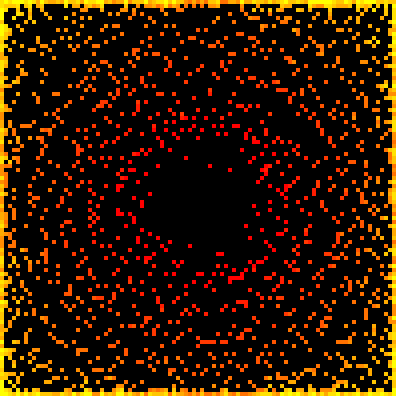### Roap Bridge

Taken from 2022 Day 9 - Road Bridge.

Here we use `matplotlib` and `imageio` to render an animation:

``````    def _init_plt(self):
""" Generate a Figure and Axes objects which are reused. """
my_dpi = 120
figure, axes = plt.subplots(figsize=(1024/my_dpi, 768/my_dpi), dpi=my_dpi, facecolor="white") # set size in pixels
axes.set_aspect('equal') # set x and y to equal aspect
axes.set_facecolor('xkcd:black')

return figure, axes

def _render_frame(self, visited: set[Point], iteration: int=0):
""" Only renders an animation frame if we've attached an enabled Animator """

fig, axes = self._plt_info
axes.clear()

# The grid will grow as the rope heads moves around
max_x = max(self._all_head_locations, key=lambda point: point.x).x
min_x = min(self._all_head_locations, key=lambda point: point.x).x
max_y = max(self._all_head_locations, key=lambda point: point.y).y
min_y = min(self._all_head_locations, key=lambda point: point.y).y
axes.set_xlim(min_x - 2, max_x + 2)
axes.set_ylim(min_y - 2, max_y + 2)

# dynamically compute the marker size
fig.canvas.draw()
factor = 40  # Smaller factor means smaller markers
mkr_size = int((axes.get_window_extent().width / (max_x-min_x+1) * (factor/fig.dpi)) ** 2)

# make sure the ticks have integer values
axes.xaxis.set_major_locator(MaxNLocator(integer=True))

tail = self._knots[-1]
others_knots = self._knots[1:-1]

visited_x = [point.x for point in visited if point != tail]
visited_y = [point.y for point in visited if point != tail]

for knot in others_knots:
axes.scatter(knot.x, knot.y, marker=MarkerStyle("."), s=mkr_size/2, color="white")